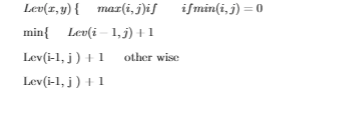# Latex Math equation format going wrong

I am trying to write the following equation into latex but it bugs out. I have linked what i am trying to, the result of it in latex and the code.

Result:Code:

Lev(x,y)\begin{cases}
& max(i,j){  if } \qquad  if min(i,j) = 0\\
& min\begin{cases}
& \text{ Lev(i-1, j ) + 1  }  \quad  \\
& \text{ Lev(i-1, j ) + 1  }  \quad  other wise\\
& \text{ Lev(i-1, j ) + 1  }  \quad  \\
\end{cases}
\end{cases}

• Welcome to TeX.SX! Works for me. Did you properly enter math-mode (surround it by $your code here$)? – TeXnician Apr 14 '18 at 7:18

Something like this?

Aside: Note the use of \min and \max as well as the definition of \Lev as a "math operator".\documentclass{article}
\usepackage{amsmath}
\DeclareMathOperator{\Lev}{Lev}
\begin{document}
$\Lev(x,y)= \begin{cases} \max(i,j) & \text{if \min(i,j) = 0}\\[1ex] \min\begin{cases} \Lev(i-1, j ) + 1 \\ \Lev(i-1, j ) + 1 \\ \Lev(i-1, j ) + 1 \end{cases} & \text{otherwise} \end{cases}$
\end{document}